# Team:ZJU-China/Model/first

Description
Light&Logic Gate Model

Overview

As our design presents, our simple cipher machine includes a light-input and light-output system. According to the reference, we have found that the intensity of GFP/RFP fluorescence is related to the intensity of the input red/green light(Ig,Ir ) the concentration of the induce substance, which is directly related to the GFP output fluorescence.
Therefore, we attempt to explore the quantitative relationship between them.
According to our design, we have combined our light-input system CcaS/R and logic AND gate system, one of whose input is a type of induces substance while the other one is controlled by light. With the change of our code book, different input substances and output substances are applied. Thus, to simplify the problem, we divide the whole model into to correlated parts, light-input and AND logic gate and build the model for only one condition, which is shown as following.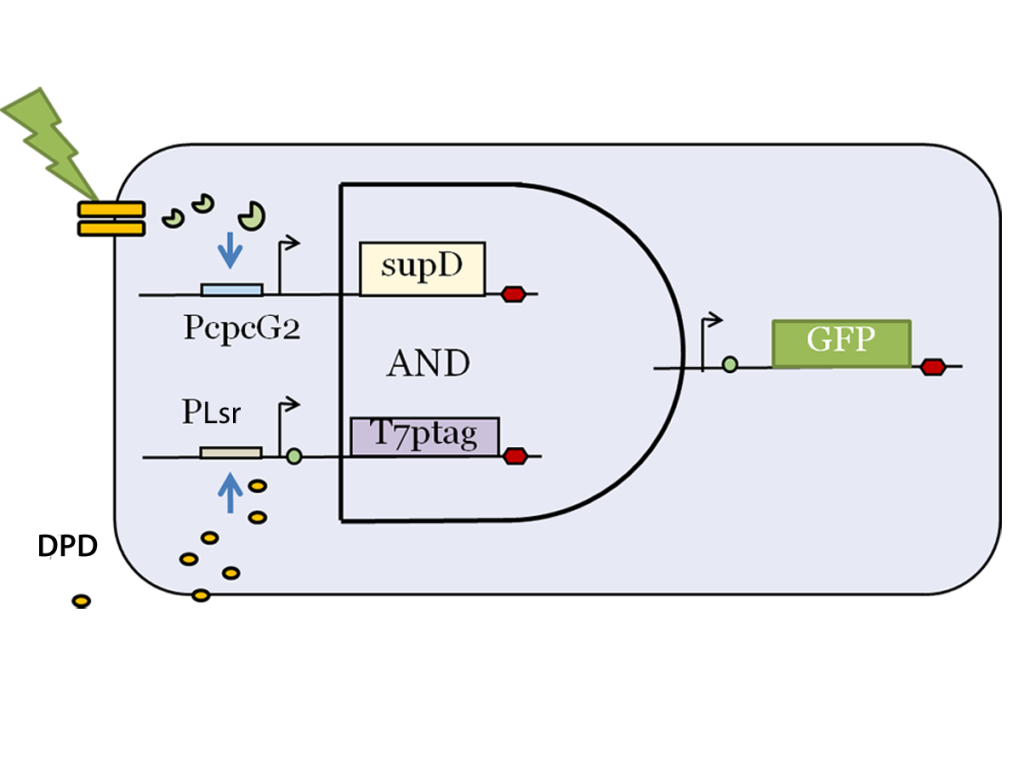Pic 1 The Modeling system

Light-Controlled Model
In our light-input part, we tried to find the relationship between the previous light intensity and the output of the Ccas/R system. According to the reference, we’ve got the following equations: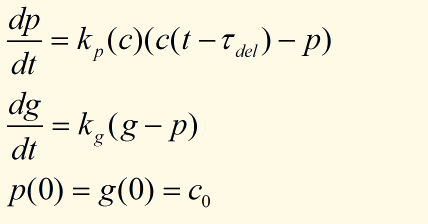The two dynamic variables in this model are the production rate of sfGFP, p(t),and the sfGFP abundance, g(t). And c0 represents the original fluorescence of sfGFP. According to our reference, the function of c(t) and kp(c) can be defined as: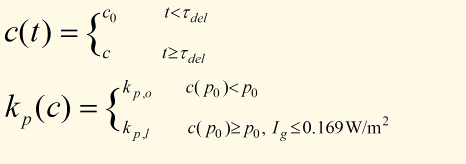Therefore, to simplify the problem, we choose to simulate the steady-state, where Ig<I0 = 0.169W/(m^2). According to our reference, if Ig>I0, the value of c will never get steady.

After applying some specific values of parameter, we test three groups of (p0, c), we can get three figures. Specifically, three groups of inputs are (17,80) and (90, 60)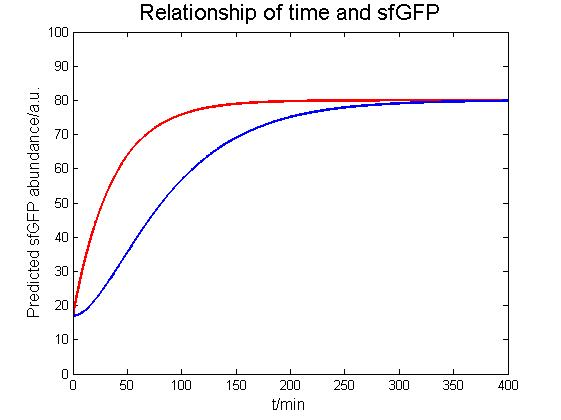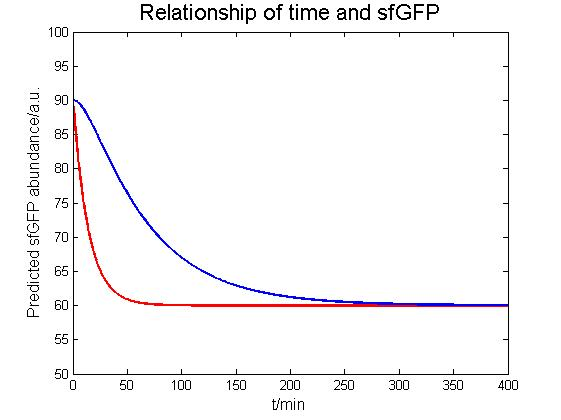Pic 2 Predicted p(t) and g(t) result

In the figure, blue line represents the p(t), while the red line represents g(t).
From the figure above we can clearly find that with time going by, both p(t) and g(t) will reach the maximum level and keep steady. Besides, the maximum value is c. The result is crucial to the derivation of the integrated model of light-input model and AND logic gate model.

AND Logic Gate Model
According to the genetic circuit of AND logic gate together with the derivation of the original reference, we have proposed the following equation by the law of mass action and Michaelis-Menten Equation. We mainly focus on the process of the output part, the production of T7 and GFP.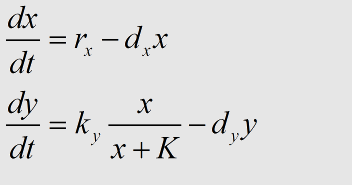Here, x(t) represents the concentration of T7 RNA polymerase, y(t) represents the concentration of GFP. rx represents the rate of production of activator protein. After our derivation, we can prove that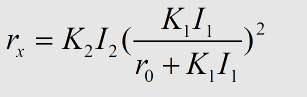where K1, K2 are the coefficient related with the concentration of T7 mRNA, I1, I2 is relative to the concentration of two input substances(In our system, Arbc.), and r0 the rate of termination of the translation.
Integrated Model
In the integrated model, we have constructed the system as Pic1 shows. For the reason that the light-input system we used, DPD-induced system has the same mechanism of CcaS/R system, so we use two crucial assumptions:
(1) The expression of the sfGFP, which is the output substance of the first gene circuit, was equal to the concentration of the input substance of the AND logic gate. That is, I1(t)=g(t) .
(2) The maximum value of g(t) is c(p0), and we get the function after the enough long time. That is, we take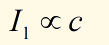when we calculate the steady state of y, which is the concentration of GFP as mentioned above.
Therefore, based on the equation , we can derive the following equation: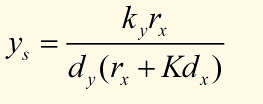where I1(t)=g(t), and K1, K2 is coefficient derived from the equation (). Because the intensity of GFP fluorescence is proportional to the concentration of GFP protein, y , we try to get the function as y=F(I1,I2,t) . However, y changes with time going by, which greatly increases the difficulty of presenting the results.
Therefore, we try to simplify the equation. We find that when t get larger , y would get closer to a steady state value, which is defined as ys , and the function can be simplified as ys=f(I1,I2) . We have demonstrated that , but I2 is still difficult to measure, so we simplify the function using the concentration of DPD. Thus, we have constructed the relationship between the two inputs (DPD and green light) and one output (GFP).
Suppose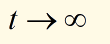, we can get the steady-state solution that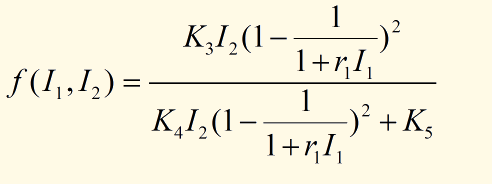Here, K3, K4, K5 are the coefficients that are related to rx, d, etc. Using MATLAB and taking different values of the coefficients, we can get the three-dimensional figure: ( Shown in Pic3)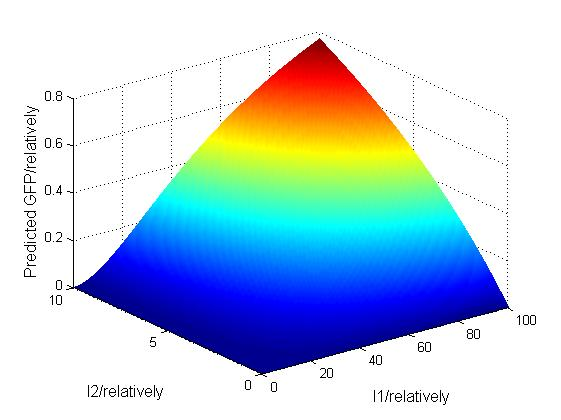Pic 3     Predicted GFP abundance of Integrated Model

From the integrated model we can conclude that our model successfully simulates the results of our light-controlled and AND logic gate experiment. Besides, Pic3 also shows the character of AND logic gate, where the system is only ON when all of the inputs are ON.